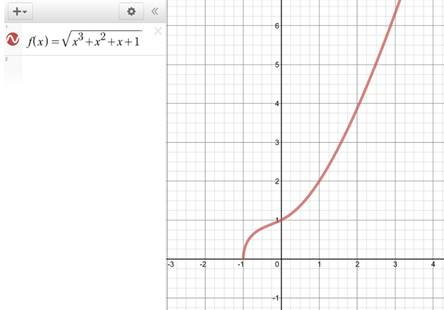# To graph: The function f ( x ) = x 3 + x 2 + x + 1 and reason for the graph to be one to one, then find an explicit expression f − 1 ( x ) .### Single Variable Calculus: Concepts...

4th Edition
James Stewart
Publisher: Cengage Learning
ISBN: 9781337687805### Single Variable Calculus: Concepts...

4th Edition
James Stewart
Publisher: Cengage Learning
ISBN: 9781337687805

#### Solutions

Chapter 1.6, Problem 57E
To determine

## To graph:The function f(x)=x3+x2+x+1 and reason for the graph to be one to one, then find an explicit expression f−1(x) .

Expert Solution

### Explanation of Solution

Consider the function.

f(x)=x3+x2+x+1

The graph of the above function is shown in figure below.Figure (1)

In the above graph, no horizontal line can be draw that intersects the graph more than once.

Thus, the function is one to one function.

To get the inverse replace the x with y and vice-versa in the given function.

x=y3+y2+y+1

With the help of CAS the solution obtained is,

y=[(1+i3)27x2+3327x440x2+16203623+23(1i3)27x2+3327x440x2+1620313]

Also,

y=[(1+i3)27x2+3327x440x2+16203623+23(1i3)27x2+3327x440x2+1620313]

And,

y=[27x2+3327x440x2+16203323+2327x2+3327x440x2+1620313]

The two different solutions are not valid because they contain i that makes them non-rea; solutions

Therefore, the inverse function is f1(x)=[27x2+3327x440x2+16203323+2327x2+3327x440x2+1620313] .

### Have a homework question?

Subscribe to bartleby learn! Ask subject matter experts 30 homework questions each month. Plus, you’ll have access to millions of step-by-step textbook answers!